# Expression of a variable from the formula - math word problems

#### Number of examples found: 808

• The ballThe ball has a radius of 2m. What percentage of the surface and volume is another sphere whose radius is 20% larger?
• Exponential warmSuppose that a body with temperature T1 is placed in surroundings with temperature T0 different from that of T1. The body will either cool or warm to temperature T(t) after time t, in minutes, where T(t)=T0 + (T1-T0)e^(-kt) . If jello salad with 30 degr
• Telegraph poles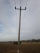The bases of two adjacent telegraph poles have a height difference of 10.5 m. How long do the wires connect the two poles if the slope is 39° 30´?
• Klara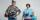Klara and Jitka went on a hiking trip at 13 o'clock at speed 5km/h. At 14 o'clock, Tomas ride on the bike at an average speed of 28 km/h. How many hours and at what distance from the beginning of the road Tomáš caught the two girls?
• Equilateral triangleA square is inscribed into an equilateral triangle with a side of 10 cm. Calculate the length of the square side.
• OrchardOne-eighth of the trees in the fruit plant in winter froze and one-twelfth of damaged disease and pests. Healthy trees remained 152. Is it enough to supply 35 trees to restore the original number of trees in the orchard?
• Painting rooms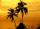If Dano paint three hours daily given work he complete in 7.5 days. How many hours a day would have to work to finish the job 1.5 days earlier?
• An observerAn observer standing west of the tower sees its top at an altitude angle of 45 degrees. After moving 50 meters to the south, he sees its top at an altitude angle of 30 degrees. How tall is the tower?
• Hexagonal pyramidCalculate the surface area of a regular hexagonal pyramid with a base inscribed in a circle with a radius of 8 cm and a height of 20 cm.
• The wellbore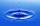The wellbore has a tributary of 2 m3 per hour. When there is no tapping, there are a stable 28 liters of water in the well. The pump suction basket is at the bottom of the well. At 14.00, the water was pumped out at a rate of 0.5 liters of water every se
• Right triangleLegs of the right triangle are in the ratio a:b = 2:8. The hypotenuse has a length of 87 cm. Calculate the perimeter and area of the triangle.
• Between two bus stops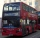Wanda lives between two bus stops at three-eighths of their distance. He started the house today and found that whether he was running to one or the other stop, he would have arrived at the bus stop. The average bus speed is 60 km/h. What is the average
• Two pipesHow long will the pool be filled with a double supply pipe if it takes the pool to fill the first pipe by 4 hours longer and the second pipe 9 hours longer than both pipes open at the same time?
• A mapA map with a scale of 1: 5,000 shows a rectangular field with an area of 18 ha. The length of the field is three times its width. The area of the field on the map is 72 cm square. What is the actual length and width of the field?
• The diagram 2The diagram shows a cone with slant height 10.5cm. If the curved surface area of the cone is 115.5 cm2. Calculate correct to 3 significant figures: *Base Radius *Height *Volume of the cone
• Two shipsThe distance from A to B is 300km. At 7 am started from A to B a ferry with speed higher by 20 km/h than a ship that leaves at 8 o'clock from B to A. Both met at 10h 24min. Determine how far they will meet from A and when they reach the destination.
• A kite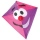Children have a kite on an 80m long rope, which floats above a place 25m from the place where children stand. How high is the dragon floating above the terrain?
• Fire tank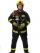Whole fire tank was discharged once in 5 days by first out by second in \$n days. Once firefighters pumped out 6/13 of water out from the tank and then let the water flow out both drain. How long take empty the tank?The quadrilateral pyramid has a rectangular base of 24 cm x 3.2dm and a body height of 0.4m. Calculate its volume and surface area.Every year, at the same time, an increase in the volume of wood in the forest is measured. The increase is regularly p% compared to the previous year. If in 10 years the volume of wood has increased by 10%, what is the number p?